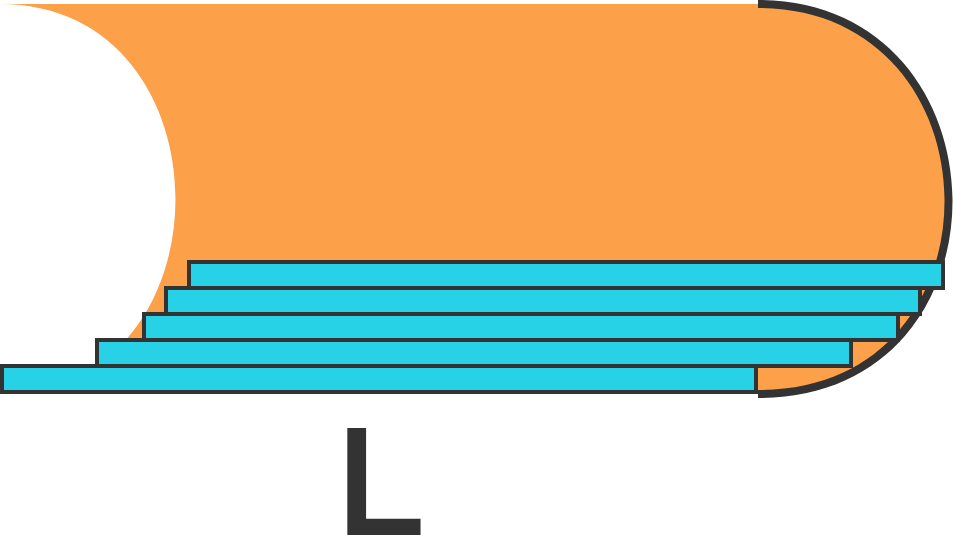# Breaking The Disc MethodThe above figure consists of a rectangle with a semicircle cut out of one end and added to the other end, where $L$ is the width of the rectangle, and the curved length of a semicircle is $\pi r$.

To calculate the area of the shaded figure, Svatejas applies the disc method as follows:Consider the axis of integration to be the semicircular arc, which has length $\pi r$. For each horizontal strip, we have an area element (technically length element) of $L$. Hence, the area is

$\int_{R} L \, dR = \pi r \times L.$

What is the area of the shaded figure?

×